# Car repair

John bought a car for a certain sum of money. He spent 10% of the cost to repairs and sold the car for a profit of Rs. 11000. How much did he spend on repairs if he made a profit of 20%?

r =  5000 Rs

### Step-by-step explanation: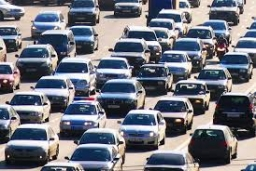Did you find an error or inaccuracy? Feel free to write us. Thank you!Tips to related online calculators
Do you have a linear equation or system of equations and looking for its solution? Or do you have a quadratic equation?

## Related math problems and questions:

• MerchantThe merchant lower cost by 10% in December when it was not sold. Again in January lower cost by 20% and now costs 576 €. A. How much the goods stood originally? B, how much cost the goods after the first lowering? C, How many percents totally merchant low
• Cost and profit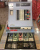Find the cost price of an article which was sold for 90.00 at a profit of 20 percent.
• The profitThe profit made by a business in 2018 was triple that made in 2019. The profit made in 2020 was R 3 million less than the profit made in 2019. If the total profit in these 3 years was R 67 million, what was the profit made in 2020?
• Profit per kiloThe trader sells apples at a profit of 20% per kilogram. Today, he decided to reduce the price of apples by 10%. How much profit does the trader have today?
• Retail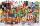At what price bought retail 1 kg goods from wholesale, if lost in the distribution is 4% of weight of the goods and retail still have a profit of 6.3%? Goods are sold at retail for 25 euro per kg.
• Profit margin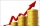If total sales for the month is $450,000 and the profit margin is 40%, how much was the cost of goods sold? • Price reductionThe product is sold for 360 CZK and the sales profit is 30%. By what percentage the sales profit will be reduced if I reduce the price of the product by 10%? • Sum of moneyOn a certain sum of money, invested at the rate of 10% compounded annually, the difference between the interests of the first year and the third year is 315 . Find the sum. • Cinema ticketsCinema sold 180 tickets this Thursday, which is 20%. Monday 14%, Tuesday 6%, Wednesday 9%, Friday 24%, Saturday 12%, and Sunday 15%. How many tickets were sold per week? • Profit growthThe profit of a company increased by 25% during the year 1992, increased by 40% during the year 1993, decreased by 20% in 1994 and increased by 10% during the year 1995. Find the average growth in the profit level over the four years periods? • Two math problems1) The sum of twice a number and -6 is nine more than the opposite of that number. Find the number. 2) A collection of 27 coins, all nickels, and dimes, is worth$2.10. How many of each coin are there? The dime, in United States usage, is a ten-cent coin.
• Dividing moneyThomas, Honza and Vasek are to divide 1220kč. Honza got 25% more than Thomas. Vasek 20% less than Thomas. How CZK each got?
• Crowns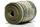1180 crowns are divided into three people: A got 20% less than B and C by 15% more than B. How many got A, B, and C?
• Sum of three numbersThe sum of three numbers from which second number is 20% smaller than the first number and the third number is 25% smaller than the second number is 96. Determine this numbers.
• Washing machineThe washing machine cost € 325. In sale-off, they became cheaper by 10%, later by another 10%. Find the last price of the washing machine.
• Car downSarah buys a car costing \$12,500. It depreciates value by 8% in the first year, 10% in the second year, and 5% in the third year. Calculate the value of a car after the third year.
• RepairingThree employees earned a total of € 469 for repairing the equipment. They split so that the first got 20% more than the second, and the third 15% more than the second. How many euros did everyone get?